# Classifying Polynomials Degree of a Polynomial The degree

• Slides: 18Classifying Polynomials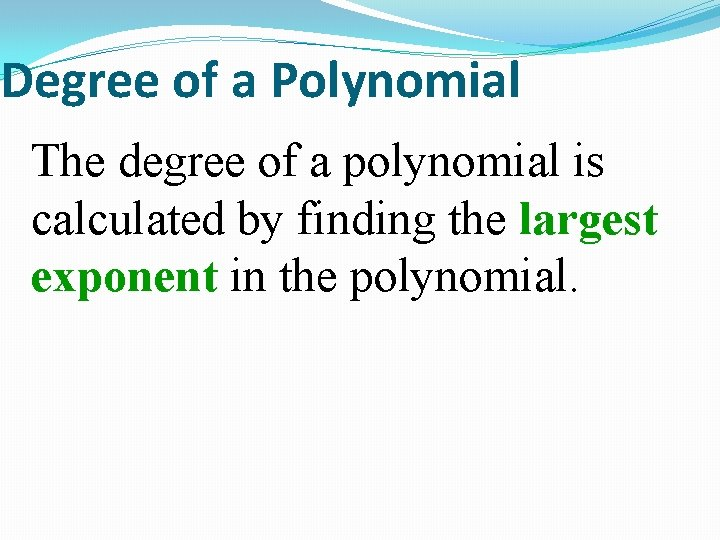Degree of a Polynomial The degree of a polynomial is calculated by finding the largest exponent in the polynomial.Degree of a Polynomial (Each degree has a special “name”) 9Degree of a Polynomial (Each degree has a special “name”) 9 No variable ConstantDegree of a Polynomial (Each degree has a special “name”) 9 8 x No variable Constant 1 st degree LinearDegree of a Polynomial (Each degree has a special “name”) 9 8 x 7 x 2 + 3 x No variable Constant 1 st degree Linear 2 nd degree Quadratic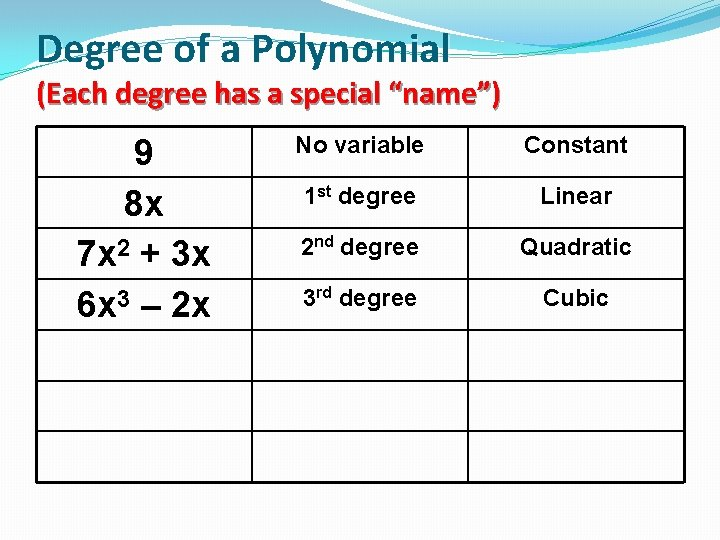Degree of a Polynomial (Each degree has a special “name”) 9 8 x 7 x 2 + 3 x 6 x 3 – 2 x No variable Constant 1 st degree Linear 2 nd degree Quadratic 3 rd degree Cubic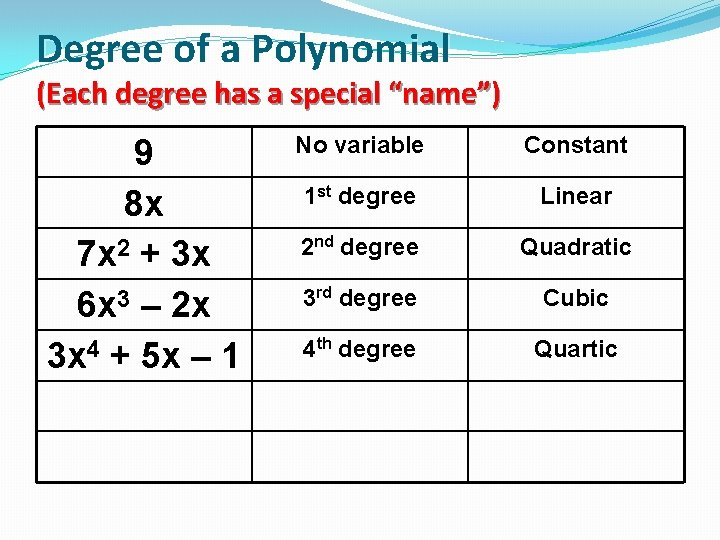Degree of a Polynomial (Each degree has a special “name”) 9 8 x 7 x 2 + 3 x 6 x 3 – 2 x 3 x 4 + 5 x – 1 No variable Constant 1 st degree Linear 2 nd degree Quadratic 3 rd degree Cubic 4 th degree QuarticDegree of a Polynomial (Each degree has a special “name”) 9 8 x 7 x 2 + 3 x 6 x 3 – 2 x 3 x 4 + 5 x – 1 2 x 5 + 7 x 3 No variable Constant 1 st degree Linear 2 nd degree Quadratic 3 rd degree Cubic 4 th degree Quartic 5 th degree QuinticDegree of a Polynomial (Each degree has a special “name”) 9 8 x 7 x 2 + 3 x 6 x 3 – 2 x 3 x 4 + 5 x – 1 2 x 5 + 7 x 3 5 xn No variable Constant 1 st degree Linear 2 nd degree Quadratic 3 rd degree Cubic 4 th degree Quartic 5 th degree Quintic 6 th degree or higher “nth” degreeLet’s practice classifying polynomials by “degree”. 1. 2. 3. 4. 5. 6. 7. 8. 9. POLYNOMIAL 3 z 4 + 5 z 3 – 7 15 a + 25 185 2 c 10 – 7 c 6 + 4 c 3 - 9 2 f 3 – 7 f 2 + 1 15 y 2 9 g 4 – 3 g + 5 10 r 5 – 7 r 16 n 7 + 6 n 4 – 3 n 2 1. 2. 3. 4. 5. 6. 7. 8. 9. DEGREE NAME Quartic Linear Constant Tenth degree Cubic Quadratic Quartic Quintic Seventh degree The degree name becomes the “first name” of the polynomial.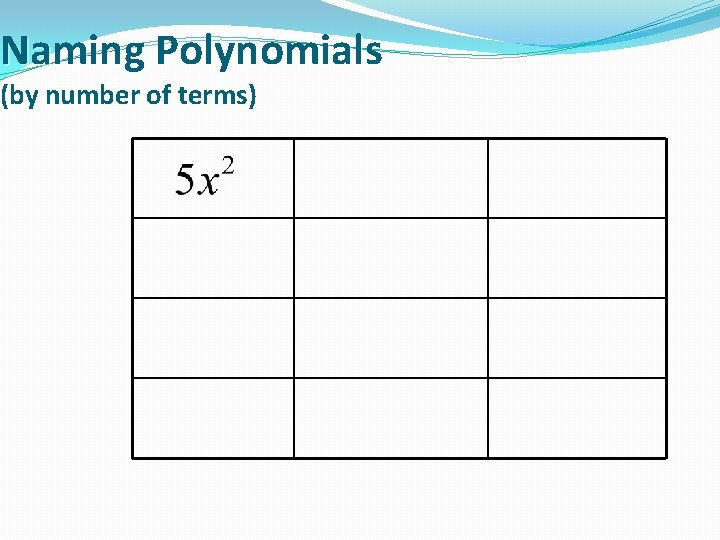Naming Polynomials (by number of terms)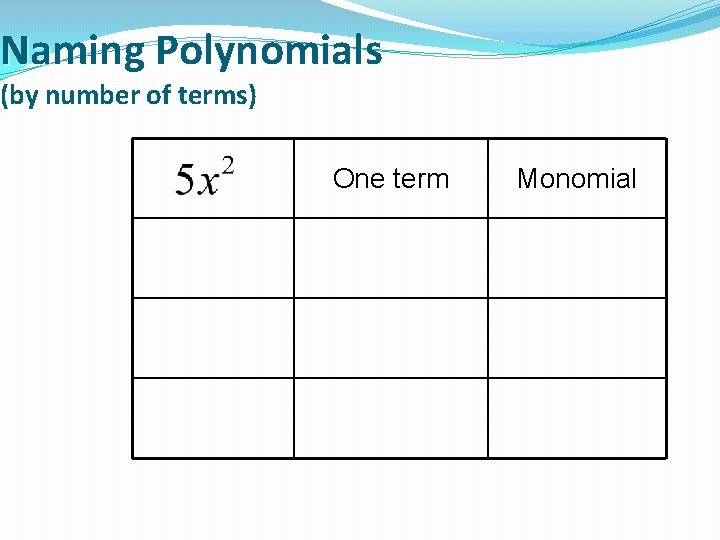Naming Polynomials (by number of terms) One term MonomialNaming Polynomials (by number of terms) One term Monomial Two terms BinomialNaming Polynomials (by number of terms) One term Monomial Two terms Binomial Three terms Trinomial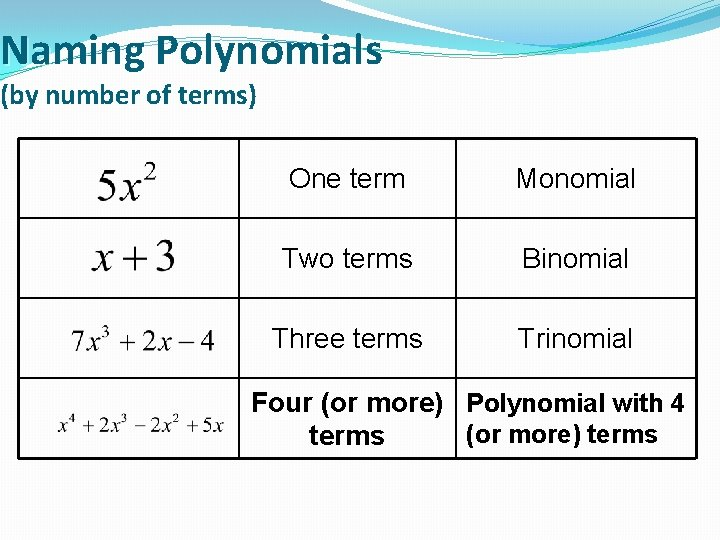Naming Polynomials (by number of terms) One term Monomial Two terms Binomial Three terms Trinomial Four (or more) Polynomial with 4 (or more) terms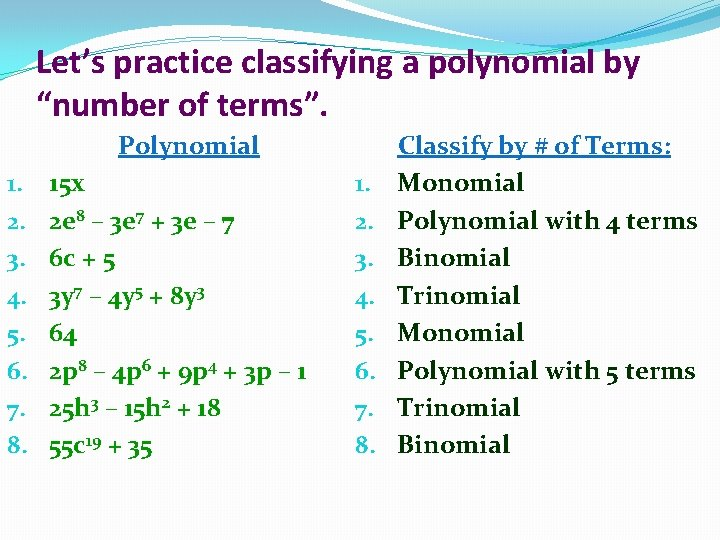Let’s practice classifying a polynomial by “number of terms”. Polynomial 1. 2. 3. 4. 5. 6. 7. 8. 15 x 2 e 8 – 3 e 7 + 3 e – 7 6 c + 5 3 y 7 – 4 y 5 + 8 y 3 64 2 p 8 – 4 p 6 + 9 p 4 + 3 p – 1 25 h 3 – 15 h 2 + 18 55 c 19 + 35 1. 2. 3. 4. 5. 6. 7. 8. Classify by # of Terms: Monomial Polynomial with 4 terms Binomial Trinomial Monomial Polynomial with 5 terms Trinomial Binomial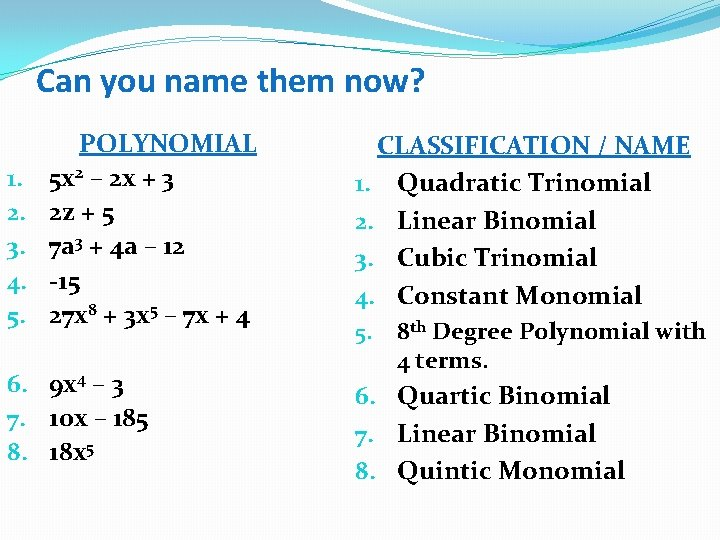Can you name them now? 1. 2. 3. 4. 5. POLYNOMIAL 5 x 2 – 2 x + 3 2 z + 5 7 a 3 + 4 a – 12 -15 27 x 8 + 3 x 5 – 7 x + 4 6. 9 x 4 – 3 7. 10 x – 185 8. 18 x 5 CLASSIFICATION / NAME 1. Quadratic Trinomial 2. Linear Binomial 3. Cubic Trinomial 4. Constant Monomial 5. 8 th Degree Polynomial with 4 terms. 6. Quartic Binomial 7. Linear Binomial 8. Quintic Monomial